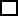Create a new printableFractions
Math Worksheets

Sample - Click above to make a new math worksheet (PDF).
 Name _____________________________Date ___________________
Number Theory
Complete.
1.   Circle all of the numbers that are divisible by 5.
 9 40 24 75 35 15
 624 5 62 72 46 132
2.   Circle all of the numbers that are divisible by 8.
 48 76 20 95 82 27
 74 72 38 42 16 15

Complete each divisibility table. Write yes if the number is divisible by the given number. Write no if it is not divisible by the given number.
3.         120
 by 2 ________ by 4 ________ by 5 ________ by 7 ________ by 10 ________
4.         144
 by 2 ________ by 3 ________ by 6 ________ by 7 ________ by 9 ________
5.         806
 by 3 ________ by 7 ________ by 9 ________ by 10 ________ by 12 ________
6.         59
 by 2 ________ by 9 ________ by 11 ________ by 12 ________ by 13 ________

Classify each number as prime or composite.
 7 83PrimeComposite
 8 35PrimeComposite
 9 16PrimeComposite
 10 85PrimeComposite
 11 29PrimeComposite
 12 99PrimeComposite
 13 32PrimeComposite
 14 92PrimeComposite

Use the clue to fill in the missing digit.
 15 The number 24is divisible by 6.
 16 The number 9is divisible by 8.
 17 The number623 is divisible by 3.
 18 The number0 is divisible by 4.
 19 The number 55 is divisible by 5.
 20 The number 568 is divisible by 7.
 21 The number 86 is divisible by 9.
 22 The number 21is divisible by 2.

 Key #2
 aKey #2
Complete.
23.   Circle all of the numbers that are multiples of 4.
 45 19 1 73 32 60
 96 29 2 20 28 53
24.   Circle all of the numbers that are multiples of 3.
 2 33 18 12 49 38
 67 1 93 85 27 87

List 3 multiples of each number.
25.
 926.
 6427.
 2728.
 529.
 7330.
 36Fill in the missing multiple.
31.
 30, ______, 50, 60, 70
32.
 ______, 12, 14, 16, 18
33.
 108, 126, 144, 162, ______
34.
 77, 88, 99, 110, ______
35.
 52, 65, 78, ______, 104
36.
 ______, 21, 28, 35, 42

List all of the factors of each number.
 37 30
 38 34
 39 84
 40 88
 41 35
 42 96
 43 13
 44 53
 45 48
 46 36
 47 57
 48 11

 Key #2
 aKey #2
Find the prime factorization of each number.
 49 12
 50 72
 51 91
 52 42
 53 77
 54 95
 55 90
 56 98
 57 85
 58 69
 59 20
 60 27

Find the greatest common factor of each set of numbers.
 61 96 and 48
 62 40 and 80
 63 46 and 72
 64 88 and 76
 65 49 and 77
 66 7 and 2
 67 80, 10, and 60
 68 60, 12, and 96
 69 54, 66, and 42

Find the least common multiple.
 70 4 and 9
 71 3 and 5
 72 6, 12, and 48
 73 3 and 9
 74 12 and 18
 75 15 and 18
 76 4, 7, and 8
 77 4, 12, and 13
 78 12 and 23

Complete.
 79 Use the digits 7, 6, and 8 to create a 3-digit number that is divisible by 2, 3, 4, 6, and 8. Describe how you found the number.
 80 The divisibility rules are helpful in math because they allow us to analyze division problems to arrive at a quick solution. The number 6 is divisible by both 2 and 3. How can you tell if a number is divisible by 6? Show by example a number that is divisible by 2, 3, and 6.